## 5.3.4 Peak Fitting

The observed peaks were asymmetric with a pronounced tail towards lower energies. After correction for detection efficiency functions of the following type [14,27,28] were fitted to every peak in energy space: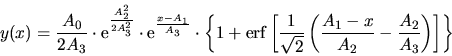(5.11)

This function is a convolution of a symmetrical function, which accounts for the straggling (gaussian distributed energy loss in front of the surface), and an asymmetrical function, which produces a tail at lower energies.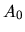,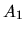,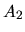, and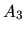are the fit parameters. The following derivation of this function type is taken from . According to  an asymmetrical peak function may be written as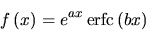(5.12)

with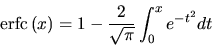(5.13)

the total area under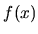equals to: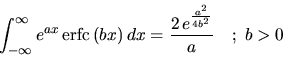(5.14)

This is used to normalize the function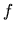to an area of 1: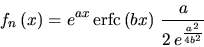(5.15)

The mean value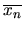and the standard deviation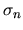are given by: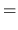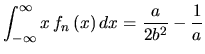(5.16)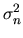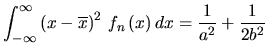(5.17)

According to  the parameters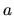and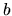are now substituted by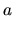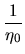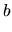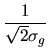(5.18)

introducing two new constants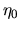and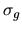. This choice will be justified below. The function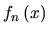may now be written as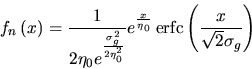(5.19)

However Equation 5.19 may also be written as a convolution of two functions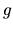and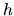normalized to 1 with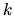being the normalization constant: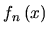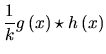(5.20)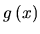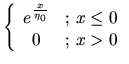(5.21)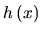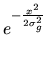(5.22)

wheredenotes the decay constant of the exponential function in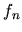andthe width of the gaussian function.

Shifting the functionalong the x-axis bysuch that the mean value is equal to 0 yields: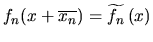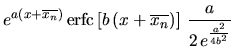(5.23)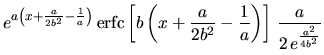(5.24)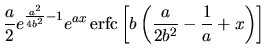(5.25)

and again substitutingand: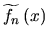(5.26)

Shifting the function so that the mean value is equal to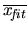(note: not) and scaled to an enclosed area of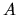yields: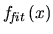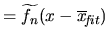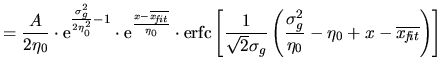(5.27)

with the following meaning of the parameters: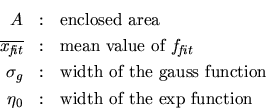The peak width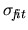of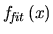is calculated using Equations 5.17 and 5.18: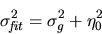(5.28)

Instead of using the mean value ofit is also possible to use the mean value of the gaussian part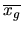ofusing Equations 5.16 and 5.18: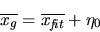(5.29)from Equation 5.27 can then be rewritten as: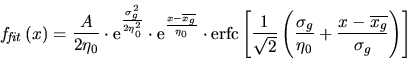(5.30)

Using the erf function instead of erfc and some transformations one obtains: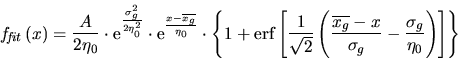(5.31)

This function is the same as that shown in Equation 5.11 with the parameters related by:

 Equation 5.11 Equation 5.31The advantage of this type of function is that values of interest such as mean value, peak width etc. may all be directly calculated from the fit parameters. As a further feature this type of function allows the separation of the symmetrical from the asymmetrical part of the fitted peak. This makes it possible to deconvolve the approximately symmetrical contribution of the energy distribution of the primary beam using the assumption that the energy distribution of the primary beam is gaussian. Additionally if molecules are used as primary particles the broadening due to dissociation can be estimated. With the definitions used in Equation 5.22 the symmetrical part can be rewritten as convolution of two gaussian functions: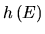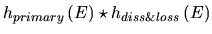(5.32)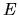denotes the energy,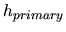the approximately gaussian distribution of the primary energy,the combined gaussian distributed energy loss in front of the surface and the broadening due to dissociation of molecules (see below for a more accurate calculation).

The width of the symmetrical part is then given by: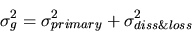(5.33)

This decomposition works well for atoms as primary particles where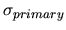is approximately half the value of. For primary molecules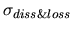dominates. In this case the gaussian approximation might no longer be valid. A simple model for the broadening due to dissociation is presented in the next chapter but it is clear that these simple calculations have their limitations.

March 2001 - Martin Wieser, Physikalisches Institut, University of Berne, Switzerland